# GMAT Practice Problems: Variables in the Answer Choices

1) Jennifer can buy watches at a price of B dollars per watch, which she marks up by a certain percentage before selling. If she makes a total profit of T by selling N watches, then in terms of B and T and N, what is the percent of the markup from her buy price to her sell price?

(A) 100T/(NB)
(B) TB/(100N)
(C) 100TN/B
(D) ((T/N) – B)/(100B)
(E) 100(T – NB)/N

2) At the store, Sam bought a shirt and a toaster.  There was an 8% sales tax on each item, and with tax, Sam paid a total of K.  If the price of the toaster before tax was T, what, in terms of K and T, is the price of the shirt before tax?

(A) 0.92(K – T)
(B) 0.92K – T
(C) 0.92(K – 1.08T)
(D) (K – T) /1.08
(E) (K/1.08) – T

3) Before January, the price of a dress was D and the price of a matching pair of shoes was H.  In January, the price of the dress increased by 40% and the price of the shoes increased by 50%.  In March, Roberta bought both items with a 30% discount.  If D = 5H, which of the following represents the amount that Roberta paid?

(A) D + 40
(B) D + H – 1
(C) D + 2H
(D) 5.95H
(E) 1.21D

4) A marketer bought N crates of empty cardboard gift boxes.  Each crate held Q individual gift boxes, and the lot of N crates was purchases at a wholesale price of W dollars.  This marketer will sell collections of J cardboard gift boxes to retailers, at a price of P dollars for each collection.  (Note: J is a divisor of Q.)  The marketer knows that, when he has sold all the cardboard gift boxes this way, he wants to net a total profit of Z dollars on the entire transaction.  What price P must he charge, to net this profit? Express P in terms of N, Q, W, J, and Z.5) In the rectangle above, the ratio of (length):(height) = A, which is a number greater than one.  Which of the following expresses the ratio of (diagonal):(length) in terms of A?6) In the diagram above, BF is an altitude drawn to the base AC, and AC is the side of square ACDE.  If BF = h, and the area of triangle ABC equals Q, find the area of the square (shaded) in terms of h and Q.Solutions are given below.

## Variables in the Answer Choices

The GMAT loves these question, not least because so many real-world situations something of this feel: we have a few changing variables (number of items sold, or stock price, or commodity price, etc.) and we want to figure out something else that depends on these changing quantities.

There are two basic approaches: an algebraic approach vs. a numerical approach.  Each one has advantages and disadvantages.  Ultimately, you should practice both approaches on a number of these problems, to get a sense of what comes most naturally for you.

If you know how to do the algebra, it is efficient and it always leads to an unambiguous answer choice.  If you can do the algebra, that’s great, but the disadvantage is that it’s sometimes hard to know how to perform this approach.

Picking numbers is inefficient but easier.   It important to know that there is an art to picking numbers.   Of course, if I pick a set of numbers for the variables in the prompt, and an answer choice doesn’t produce the desired answer, I can eliminate it, but if these numbers do produce the desired answer, I can draw no conclusion from that.  Picking numbers is, de facto, a process of elimination approach.   We have to pick numbers until four answer choices have been clearly eliminated.  Obviously, the test loves to design questions so that the most obvious choices for numbers eliminate few or no answers.  Each round of picking numbers is simple and relatively quick, but when you begin, you have no idea how many rounds it will take before four answer choices have been eliminated.  That’s the inefficient part.

In the problems below, I will demonstrate both approaches for each problem, and in demonstrating the numerical approach, I will discuss in context some points of strategy for picking numbers.

## Summary

If you have been working with these problems, and have your own thoughts about the algebraic approach vs. the picking-numbers approach, we would love to hear from you.  Let us know in the comment section below!## Practice problem explanations

As you read these explanations, pay attention to which solution seems more natural and more doable for you.

1) Algebraic Solution: If she makes a total profit of T for N watches, then that must be a profit of T/N for each watch.  That must be the markup above cost on each watch, the amount of the increase.  Well, percent increase = (amount of increase)/(starting amount) x 100% = (T/N)/B *100 = 100T/(NB)

That Algebraic solution was elegant if you saw it, but if not, here’s a full solution with picking numbers.

Numerical Solution: The price of each watch, I will pick B = 7, a prime number.   For the percent increase, I will make this easy.  Picking 100% is too easy, and too predictable, so I am going to pick 400% — 400% of 7 is 28, and the \$7 watches are marked up \$25, then the selling price is \$35.  The profit per watch is \$28, and so if she sells N = 11 watches (another prime number), that would be a profit of T = 28*11 = \$308.  Leaving T in unmultiplied form will make it easier to cancel.

OK, now we will plug in T = 308 = 11*28, N = 11, and B = 7, and hope to get 400 as our answer.

(A) 100T/(NB) = 100*11*28/(11*4) = 100*28/7 = 110*4 = 400 = works!

(B) TB/(100N) = 11*28*7/(100*11) = 28*7/100 = doesn’t work!

(C) 100TN/B = 100*11*28*11/7 = 100*11*4*11 = doesn’t work!

(D) ((T/N) – B)/(100B) = [(11*28/11) – 7]/(7*100) = (28 – 7)/(7*100) = 21/(7*100) = 3/100 = doesn’t work

(E) 100(T – NB)/N = 100(11*28 – 11*7)/7 = 100*11*21/7 = 100*11*3 = doesn’t work!

We were lucky here.  With one choice of numbers, we were able to eliminate four answer choices, leaving (A) as the only possible answer.

2) Algebraic Solution: Call the price of the shirt S.  The price of the toaster is T.  The total before tax is (S + T).  Then an 8% tax was applied.  For an 8% increase, we use the multiplier 1.08.  Thus, K = 1.08*(S + T). Solve this for S.

S + T = K/1.08

S = K/1.08 – T

Numerical Solution: we make our lives much easier if we first recognize the percent trap.  The multiplier 1.08 is the correct multiplier for an 8% increase, and it makes sense that this would appear somehow in the correct answer.   BUT, 0.92 is the multiplier for an 8% decrease.  This is tricky: an 8% decrease is not the opposite of an 8% increase; it does not “undo” an 8% increase.  In other words, an 8% increase followed by an 8% decrease does NOT get us back to the original starting point.  That’s a major percent trap.  There is a 8% increase in the problem, but no 8% decrease: this means that any answer in which the multiplier for an 8% decrease, 0.92, appears, it is automatically wrong.   We can immediately eliminate (A) & (B) & (C) without plugging in anything.

OK, let’s say T = 50, shirt = 150, so total before tax is \$200. (Again, avoiding the cliché price of \$100 for a percent.)  Now, 8% of \$200 is \$16, for a total of K = 216.  Because dividing by a decimal isn’t fun, I am going to change the division by 1.08 into multiplication by the fraction 100/108.  We are looking for an answer that equals 150, the price of the shirt.

(D) (K – T) /1.08 = (216 – 50)/1.08 = 156*100/108 = 39*100/27 = 13*100/9

well, 13/9 doesn’t equal 1.5, so this doesn’t work.

(E) (K/1.08) – T = 216/1.08 – 50 = 216*(100/108) – 50 = 2*108*(100/108) – 50

= 2*100 – 50 = 200 – 50 = 150

That’s the price of the shirt!  Choice (E) is the answer!

3) Algebraic Solution: dress increase to 1.4D, and the shoes increase to 1.5H.  The multiplier for a 30% decrease is 0.7, so the total amount could be written as 0.7*(1.4D + 1.5H).  This does not appear as an answer.  Since H is smaller, write D in terms of H: D = 5H.  Then the total is 0.7*(1.4(5H) + 1.5H) = 0.7*(7H + 1.5H) = 4.9H + 1.05H = 5.95H.  That’s answer (D).

Numerical Solution: What do you think every single drooling GMAT test taker is going to pick for the price of the dress?  You guessed it: \$100 dress and \$20 shoes.  It’s a spectacularly bad idea to make that choice.  As will happen on the real GMAT, I was anticipating this choice, and composed trap answers that would work for this test.  If you plug this in, I guarantee you will not be able to eliminate four answers at once.

Think about making a choice that is still relatively easy, but not everyone would make it.

Make the dress D = 300, so H = 60.  D increased by 40% — 10% of 300 is 30, so 40% must be 4*30 = 120, and the new dress price is \$420.  The shoes increase by 50%, to \$90.  Together, after the increase, dress + shoes would cost 420 + 90 = \$510.  Now, Roberta gets a 30% decrease on this price.  Well, 10% of 510 is 51, so 30% would be 3*51 = 153.  510 – 153 = 357.  The correct answer must equal 357.

(A) D + 40 = 340 = not correct

(B) D + H – 1 = 300 + 60 – 1 = 359 = close, but no cigar!

(C) D + 2H = 300 + 2*60 = 420 = not even close

(D) 5.95H = 5.95*60 — hmmm — I am going to think of 60 as 10*2*3.  First, 59.5*10 = 59.5. Then 59.5*1 = 119.  Then 119*3 = 357.  This works.

(E) 1.21D = 1.21*300 = (1 + 0.2 + 0.01)*300 = 300 + 60 + 3 = 363 = not correct

The only possible answer is (D).

4) Algebraic Solution: So, the marketer initially paid W: that was his outlay, his cost.   There are Q boxes in each crate, and J boxes make a collection, so there are Q/J collections in each crate, and NQ/J collections in total.  If he charges a price P, his revenue would be PNQ/J.  Now, profit equals revenue minus cost, so Z = PNQ/J – W .  Solve this for PNumerical Solution: Here, we have several choices to make, and it would be a good idea to pick numbers that aren’t the same, or aren’t even divisible by each other if that’s not required. Picking some different prime numbers is good way eliminate more than one answer that comes out to the correct value.

I will say the price is 2, a nice round prime number.   Let’s say J = 3, so Q must be a multiple of that — say Q = 30.  Let’s N = 7.  This means he has 10 collections from every box, and 70 collections from the total lot.  If he charges \$2 per collection, that’s a revenue of \$140.  Let’s say his outlay was W = \$95; then his profit is Z = \$45.

OK, that’s an unusual enough set of number that we expect no more than one solution will work.  We will plug in N = 7, Q = 30, W = 95, J = 3, and Z = 45, and we hope to get an output of P = 2.Thus, because we chose numbers well, the only possible answer is (B).

5) Algebraic Solution: Let length = L, height = H, and diagonal = D.  Clearly, these three are related by the Pythagorean Theorem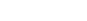Divide both sides by L squared.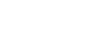The right side is the square of the answer we seek.  The left side is the square of the reciprocal of A.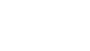Take a square root of both sides; remember that we cannot distribute the square root across addition: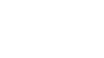Numerical Solution: Let’s choose an easy 3-4-5 triangle for the height-length-diagonal.   Then A = 4/3, and (diagonal):(length) = 5/4.  We want to plug in A = 4/3 and get an answer of 5/4.This choice of numbers eliminated four answers and leaves only one, so (E) must be the answer.

6) Algebraic Solution: Let s be the side of the square, which means it is also the base of the triangle.  We know that the area Q must equal one half times base s times height h.

Q = (1/2)sh

Solve this for s.

2Q = sh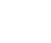Now, square this to get the area of the square: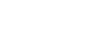Numerical Solution: Let’s say that h = 3, and that the side of the square is 8.  (Notice, I picked these so neither was a factor of the other, and I made one even so the area of the triangle Q would be a whole number.)  Then the area of the triangle is Q = (0.5)(3)(8) = 12, and the area of the square is 64.  Therefore, we should plug in h = 3 and Q = 12, and get an output of 64.This choice of numbers eliminated four answers and leaves only one, so (D) must be the answer.

## Author

•Mike served as a GMAT Expert at Magoosh, helping create hundreds of lesson videos and practice questions to help guide GMAT students to success. He was also featured as "member of the month" for over two years at GMAT Club. Mike holds an A.B. in Physics (graduating magna cum laude) and an M.T.S. in Religions of the World, both from Harvard. Beyond standardized testing, Mike has over 20 years of both private and public high school teaching experience specializing in math and physics. In his free time, Mike likes smashing foosballs into orbit, and despite having no obvious cranial deficiency, he insists on rooting for the NY Mets. Learn more about the GMAT through Mike's Youtube video explanations and resources like What is a Good GMAT Score? and the GMAT Diagnostic Test.